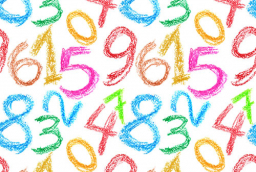# Four numbers

I am a four-digit number, no zeros, in which the first number is five times the last, the second is four more than the first and three times the third, and the third is two more than the last and two less than the first.

x =  5931

### Step-by-step explanation:Did you find an error or inaccuracy? Feel free to write us. Thank you!

Tips for related online calculators
Do you have a system of equations and looking for calculator system of linear equations?